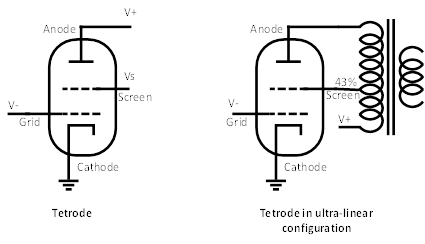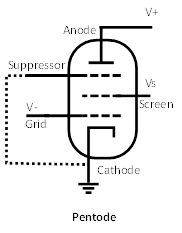The operating principle of an electronic vacuum tube is similar to that of a hydraulic valve. Electrons travel along electric wires or vacuum space, in place of water flowing in pipes. The electric potential (or voltage) forces electrons to traverse electric wires and vacuum space, in place of the potential energy due to the effect of the gravity pushing water to flow through pipes. Finally, an electronic valve can control the flow of electrons, analogously to what described for hydraulic valves.

In the following sections, we will introduce the basic types of electronic vacuum tubes and their operating principles.

### 2.2.1    Diode

The simplest type of electronic vacuum tube is the diode, depicted on the left of Figure 3. A diode has two terminals: a cathode and an anode. When the cathode is sufficiently heated, electrons start to leave its surface, due to the thermionic effect. When an electric potential V+, higher than that of the cathode, is applied to the anode, the electrons emitted by the cathode, which have a negative charge, are attracted and move toward the anode itself.

By convention, the direction of the electric current is that of the “positive” charges. Therefore, even if the electrons move from the cathode to the anode, we say that the electric current goes from the anode to the cathode. In fact, a current of negative charges in one direction is equivalent to a current of positive charges in the opposite direction.Figure 3: Diode and triode.Diode, on the left. Triode on the right. When the cathode of a vacuum tube is heated, electrons are emitted. If the anode has an electric potential higher than the cathode, the emitted electrons are attracted by it and an electric current start to flow (by convention the current goes from the positive to the negative end, even if the electrons, which have a negative charge, move from the negative to the positive end). In a triode, the negative electric potential of the grid, with respect to the cathode, can be used to control the flow of electrons from the cathode to the anode.

### 2.2.2    Triode

What we have just described above is very similar to the example we made in Section 2.1, where the water flows from the bucket through the pipe under the effect of the gravity. Here the electric current flows from the high potential V+, applied to the anode, toward the ground potential of the cathode. However, a valve that allows controlling this flow is still needed.

In a triode, as depicted on the right of Figure 3, a third terminal, called grid, is placed between the anode and the cathode. When the electric potential of the grid is lower than that of the cathode, the electrons emitted by the cathode are repelled and find difficulties to reach the anode. If the grid is negative enough, current is blocked, obtaining the same effect of closing the valve in the hydraulic circuit. If the grid potential is the same than that of the cathode, the current flows freely from the anode to the cathode, obtaining the same effect of fully opening the valve in the hydraulic circuit. Intermediate negative grid voltages modulate the current flowing from the anode to the cathode . When a voltage signal is applied to the grid, the electric current, from the anode to the cathode, follows the signal applied to the grid.

It is important to note that no current traverses the grid in normal operations. Being the grid negative, with respect to the cathode, it repels electrons and there is no electric current.

### 2.2.3    Tetrode

The basic idea of the triode was refined with the introduction of the tetrode. A tetrode, as shown in Figure 4 on the left, has a fourth electrode called screen, between the anode and the grid. The screen has the purpose of reducing the capacitance produced by the grid and the anode. In fact, in a triode, the grid and the anode are very close one to the other and jointly behave as a small capacitor, which might cause instability and oscillations. If the screen has a voltage higher than that of the cathode and the grid, but lower than the anode, it acts as an electrostatic screen between the grid and the anode, thus reducing their inherent capacitance.Figure 4: Tetrode.In a tetrode, on the left, a fourth electrode is used as a screen to limit the inherent capacitance between grid and anode. The screen can also be set to operate in ultra-linear configuration, on the right, by providing it with a percentage of the anode output signal.

### 2.2.4    Tetrode in ultra-linear configuration

In a tetrode, the screen is positive with respect to the cathode. Thus, it attracts a certain amount of the electrons emitted from the cathode itself, which would have gone to the anode. The result is a small current flowing through the screen. This effect is exploited to configure a vacuum tube to work in distributed load, or ultra-linear modality. This configuration is obtained by feeding back a percentage of the anode output signal to the screen, rather than applying a fixed voltage to it. The wanted percentage of anode output signal is typically provided to the screen by connecting it to a tap, coming out from the output transformer, as shown on the right side of Figure 4. The current through the screen, produces a sort of negative feedback and, with the appropriate percentage of anode signal, distortion falls at very small values, just slightly reducing power efficiency. The optimal percentage to be applied to the screen depends on the specific electronic vacuum tubes used. In many power amplifier designs, this percentage is generally set around 43%.

### 2.2.5    Pentode

Tetrodes were further refined with the introduction of the pentode. When electrons emitted by the cathode reach the anode, they might have enough energy to stimulate secondary electron emission from the anode itself. Secondary emission electrons might reach the screen grid, causing instability and oscillations. In order to prevent secondary emission electrons from reaching the screen grid, pentodes use a fifth electrode, called suppressor, as shown in Figure 5. The suppressor is generally directly connected to the cathode, either with an internal connection in the vacuum tube, or by an explicit connection between the corresponding pins, as depicted by the dashed line in Figure 5.Figure 5: Pentode. In a pentode, a fifth electrode, called suppressor is added to attract secondary emission electrons, emitted by the anode when hit by electrons arriving from the cathode. This prevents secondary emission electrons from reaching the screen grid, causing instability and oscillations.